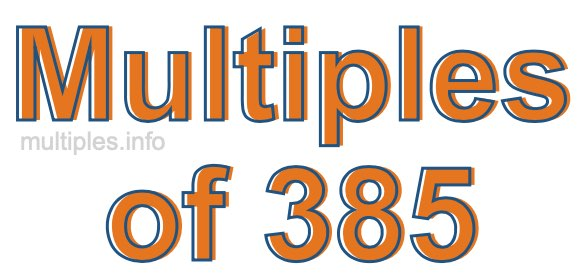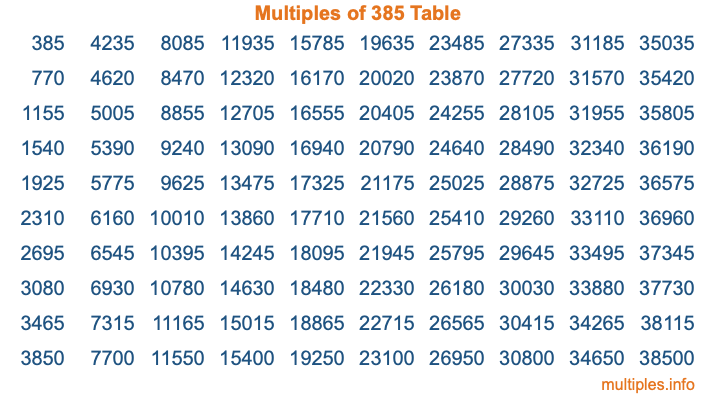Multiples of 385Welcome to the Multiples of 385 page. Here we will first teach you everything you will ever need to know about the multiples of 385, and then give you a study guide summary of everything we taught you to make sure you remember it all. Use this page to look up facts and learn information about the multiples of 385. This page will make you a multiples of three hundred eighty-five expert!

Definition of Multiples of 385
Multiples of 385 are all the numbers that when divided by 385 equal an integer. Each of the multiples of 385 are called a multiple. A multiple of 385 is created by multiplying 385 by an integer.

Therefore, to create a list of multiples of 385, you start with 1 multiplied by 385, then 2 multiplied by 385, then 3 multiplied by 385, and so on for as long as you want. Thus, the list of the first five multiples of 385 is 385, 770, 1155, 1540, and 1925. To see a larger list of multiples of 385, see the printable image of Multiples of 385 further down on this page. We also have a category where you can choose any nth multiple of 385.

Multiples of 385 Checker
The Multiples of 385 Checker below checks to see if any number of your choice is a multiple of 385. In other words, it checks to see if there is any number (integer) that when multiplied by 385 will equal your number. To do that, we divide your number by 385. If the the quotient is an integer, then your number is a multiple of 385.

Is  a multiple of 385?

Least Common Multiple of 385 and ...
A Least Common Multiple (LCM) is the lowest multiple that two or more numbers have in common. This is also called the smallest common multiple or lowest common multiple and is useful to know when you are adding our subtracting fractions. Enter one or more numbers below (385 is already entered) to find the LCM.

Check out our LCM Calculator if you need more details about the Least Common Multiple or if you need the LCM for different numbers for adding and subtraction fractions.

nth Multiple of 385
As we stated above, 385 is the first multiple of 385, 770 is the second multiple of 385, 1155 is the third multiple of 385, and so on. Enter a number below to find the nth multiple of 385.

th multiple of 385

Multiples of 385 vs Factors of 385
385 is a multiple of 385 and a factor of 385, but that is where the similarities end. All postive multiples of 385 are 385 or greater than 385. All positive factors of 385 are 385 or less than 385.

Below is the beginning list of multiples of 385 and the factors of 385 so you can compare:

Multiples of 385: 385, 770, 1155, 1540, 1925, etc.

Factors of 385: 1, 5, 7, 11, 35, 55, 77, 385

As you can see, the multiples of 385 are all the numbers that you can divide by 385 to get a whole number. The factors of 385, on the other hand, are all the whole numbers that you can multiply by another whole number to get 385.

It's also interesting to note that if a number (x) is a factor of 385, then 385 will also be a multiple of that number (x).

Multiples of 385 vs Divisors of 385
The divisors of 385 are all the integers that 385 can be divided by evenly. Below is a list of the divisors of 385.

Divisors of 385: 1, 5, 7, 11, 35, 55, 77, 385

The interesting thing to note here is that if you take any multiple of 385 and divide it by a divisor of 385, you will see that the quotient is an integer.

Multiples of 385 Table
Below is an image of the first 100 multiples of 385 in a table. The table is in chronological order, column by column. The first column has the first ten multiples of 385, the second column has the next ten multiples of 385, and so on.The Multiples of 385 Table is also referred to as the 385 Times Table or Times Table of 385. You are welcome to print out our table for your studies.

Negative Multiples of 385
Although not often discussed or needed in math, it is worth mentioning that you can make a list of negative multiples of 385 by multiplying 385 by -1, then by -2, then by -3, and so on, to get the following list of negative multiples of 385:

-385, -770, -1155, -1540, -1925, etc.

Multiples of 385 Summary
Below is a summary of important Multiples of 385 facts that we have discussed on this page. To retain the knowledge on this page, we recommend that you read through the summary and explain to yourself or a study partner why they hold true.

There are an infinite number of multiples of 385.

A multiple of 385 divided by 385 will equal a whole number.

385 divided by a factor of 385 equals a divisor of 385.

The nth multiple of 385 is n times 385.

The largest factor of 385 is equal to the first positive multiple of 385.

385 is a multiple of every factor of 385.

385 is a multiple of 385.

A multiple of 385 divided by a divisor of 385 equals an integer.

385 divided by a divisor of 385 equals a factor of 385.

Any integer times 385 will equal a multiple of 385.

Multiples of a Number
Here you can get the multiples of another number, all with the same attention to detail as we did for multiples of 385 on this page.

Multiples of
Multiples of 386
Did you find our page about multiples of three hundred eighty-five educational? Do you want more knowledge? Check out the multiples of the next number on our list!